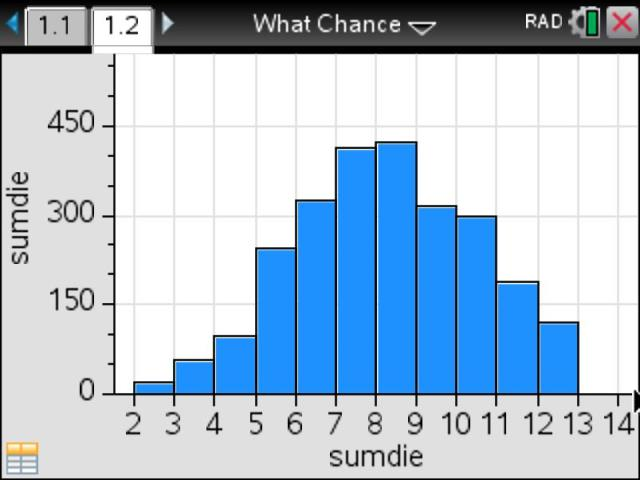# Activities

•• ##### Subject Area

• Standard: National Curriculum 7-10: Statistics and Probability: Chance

• ##### AuthorMiddle

90 Minutes

• ##### Device
•TI-Nspire™ CX
•TI-Nspire™ CX CAS
• ##### Software

TI-Nspire™
TI-Nspire™ CAS

4.2

• ##### Report an Issue

What are the Chances?#### Activity Overview

This task is designed to familiarise students with random number generators and their use in simulating chance events. It is suitable for students in Years 9 ‑ 11.

#### Objectives

• ACMSP226 – Calculate relative frequencies from given or collected data to estimate probabilities of events involving 'and' or 'or' .
• Use of a random number generator to simulate chance events
• Linking experimental relative frequencies with theoretical probabilities

#### Vocabulary

random integer, relative frequency, theoretical probability, simulation

#### About the Lesson

Part 1 - Focuses on individual trials of the rolling of a die.
Part 2 - Focuses on generating results for a larger number of trials, and the use of count commands and histograms as tools for analysing these results. It also introduces relative frequency as an approximation to the theoretical probability of a particular outcome.
Part 3 - Introduces the theoretical probability via the sum of two-dice experiment. Students compare the theoretical values with the relative frequencies obtained via the simulation.